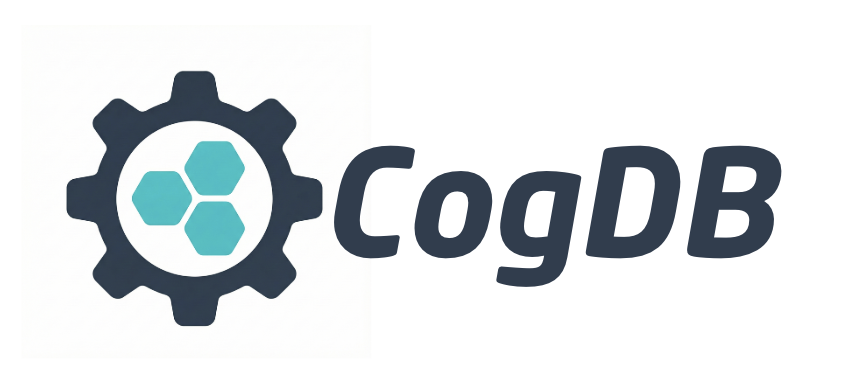#cogdb.io

New release: 2.0.5!## Installing Cog

``````pip install cogdb
``````

Cog is a persistent embedded graph database implemented purely in Python. Torque is Cog's graph query language. Cog also provides a low level API to its fast persistent key-value store.

Cog is ideal for python applications that does not require a full featured database. Cog can easily be used as a library from within a Python application. Cog be used interactively in an IPython environment like Jupyter notebooks.

Cog can load a graph stored as N-Triples, a serialization format for RDF. See Wikipedia, W3C for details.

In short, an N-Triple is sequence of subject, predicate and object in a single line that defines a connection between two vertices:

`vertex <predicate> vertex`

Learn more about RDF triples

### Creating a graph

```from cog.torque import Graph
g = Graph("people")
g.put("alice","follows","bob")
g.put("bob","follows","fred")
g.put("bob","status","cool_person")
g.put("charlie","follows","bob")
g.put("charlie","follows","dani")
g.put("dani","follows","bob")
g.put("dani","follows","greg")
g.put("dani","status","cool_person")
g.put("emily","follows","fred")
g.put("fred","follows","greg")
g.put("greg","status","cool_person")
```

### Create a graph from CSV file

```from cog.torque import Graph
g = Graph("books")
g.load_csv('test/test-data/books.csv', "isbn")
```

### Torque query examples

#### Scan vertices

```g.scan(3)
```

{'result': [{'id': 'bob'}, {'id': 'emily'}, {'id': 'charlie'}]}

#### Scan edges

```g.scan(3, 'e')
```

{'result': [{'id': 'status'}, {'id': 'follows'}]}

#### Starting from a vertex, follow all outgoing edges and list all vertices

```g.v("bob").out().all()
```

{'result': [{'id': 'cool_person'}, {'id': 'fred'}]}

#### Everyone with status 'cool_person'

```g.v().has("status", 'cool_person').all()
```

{'result': [{'id': 'bob'}, {'id': 'dani'}, {'id': 'greg'}]}

#### Include edges in the results

```g.v().has("follows", "fred").inc().all('e')
```

{'result': [{'id': 'dani', 'edges': ['follows']}, {'id': 'charlie', 'edges': ['follows']}, {'id': 'alice', 'edges': ['follows']}]}

#### starting from a vertex, follow all outgoing edges and count vertices

```g.v("bob").out().count()
```

'2'

### See who is following who and create a view of that network

#### Note: `render()` is supported only in IPython environment like Jupyter notebook otherwise use view(..).url.

By tagging the vertices 'from' and 'to', the resulting graph can be visualized.

```g.v().tag("from").out("follows").tag("to").view("follows").render()

```

#```g.v().tag("from").out("follows").tag("to").view("follows").url

```

file:///Path/to/your/cog_home/views/follows.html

#### List all views

``````g.lsv()
``````

['follows']

#### Load existing visualization

``````g.getv('follows').render()
``````

#### starting from a vertex, follow all out going edges and tag them

```g.v("bob").out().tag("from").out().tag("to").all()
```

{'result': [{'from': 'fred', 'id': 'greg', 'to': 'greg'}]}

#### starting from a vertex, follow all incoming edges and list all vertices

```g.v("bob").inc().all()
```

{'result': [{'id': 'alice'}, {'id': 'charlie'}, {'id': 'dani'}]}

## Loading data from a file

#### Triples file

```from cog.torque import Graph
g = Graph(graph_name="people")
g.load_triples("/path/to/triples.nt", "people")
```

#### Edgelist file

```from cog.torque import Graph
g = Graph(graph_name="people")
g.load_edgelist("/path/to/edgelist", "people")
```

## Low level key-value store API:

Every record inserted into Cog's key-value store is directly persisted on to disk. It stores and retrieves data based on hash values of the keys, it can perform fast look ups (O(1) avg) and fast (O(1) avg) inserts.

```from cog.database import Cog

cogdb = Cog('path/to/dbdir')

# create a namespace
cogdb.create_namespace("my_namespace")

# create new table
cogdb.create_table("new_db", "my_namespace")

# put some data
cogdb.put(('key','val'))

# retrieve data
cogdb.get('key')

# put some more data
cogdb.put(('key2','val2'))

# scan
scanner = cogdb.scanner()
for r in scanner:
print r

# delete data
cogdb.delete('key1')

```

## Config

If no config is provided when creating a Cog instance, it will use the defaults:

``````COG_PATH_PREFIX = "/tmp"
COG_HOME = "cog-test"
``````

### Example updating config

```from cog import config
config.COG_HOME = "app1_home"
data = ('user_data:id=1','{"firstname":"Hari","lastname":"seldon"}')
cog = Cog(config)
cog.create_namespace("test")
cog.create_table("db_test", "test")
cog.put(data)
scanner = cog.scanner()
for r in scanner:
print r

```

## Performance

Put and Get calls performance:

put ops/second: 18968

get ops/second: 39113

The perf test script is included with the tests: insert_bench.py and get_bench.py

INDEX_LOAD_FACTOR on an index determines when a new index file is created, Cog uses linear probing to resolve index collisions. Higher INDEX_LOAD_FACTOR leads slightly lower performance on operations on index files that have reached the target load.

#### Put and Get performance profile

Get A Weekly Email With Trending Projects For These Topics
No Spam. Unsubscribe easily at any time.
python (54,408
library (1,330
database (1,239
graph (403
nosql (177
key-value (86
graph-database (75
embedded-database (22
hashtable (20
network-graph (18